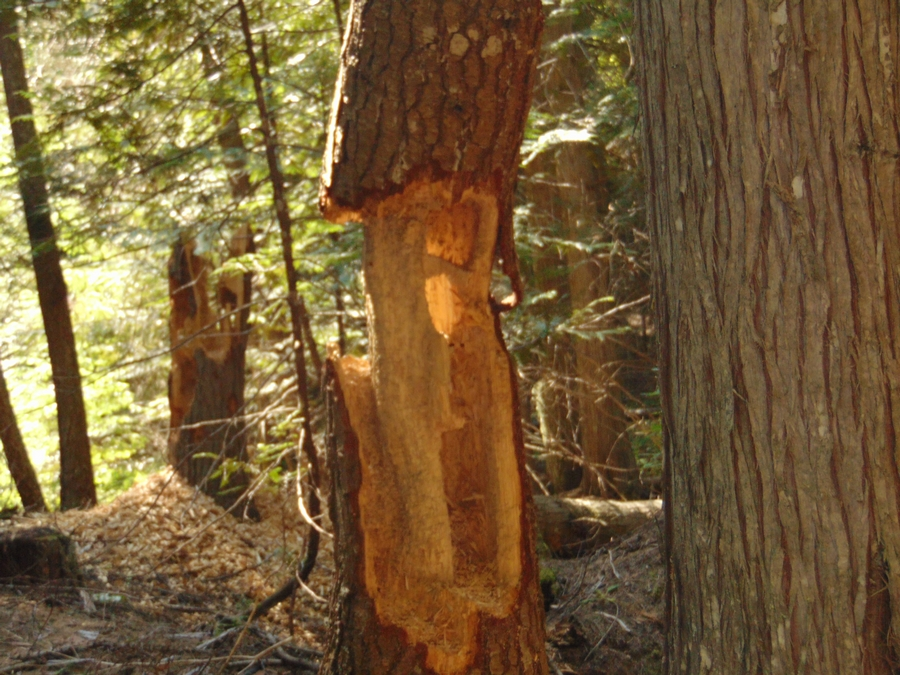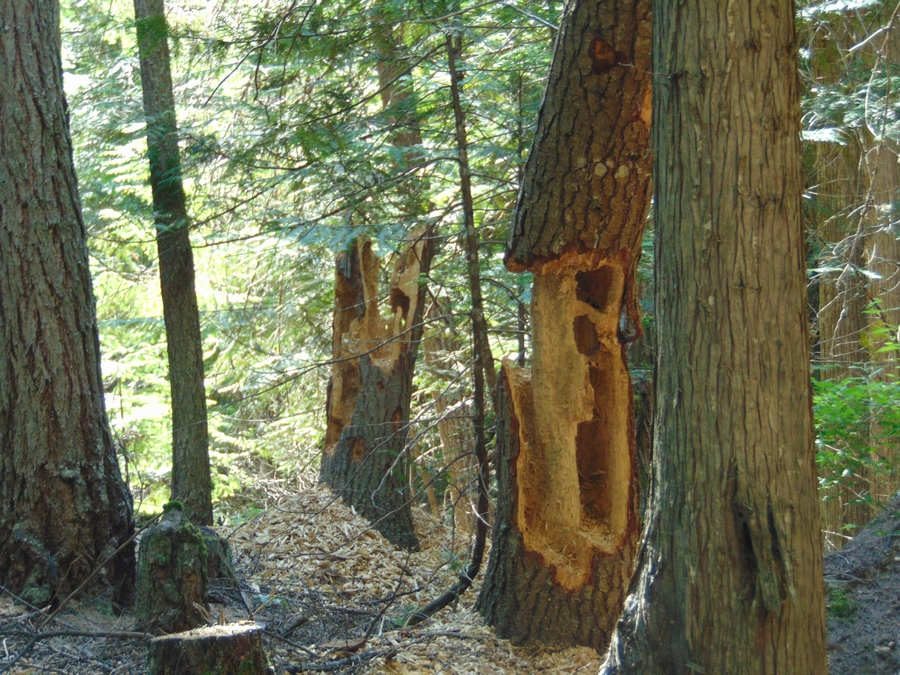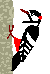this tree died a few years back and woodpeckers turned it into this pretty cool looking 'art piece' (in the background is another tree that woodpeckers had a go of too)
unfortunately these trees are down a very long and very steep pathway where the 3-wheeler wouldnt be able to make the trek back up with the weight of even half a load of wood let alone a full load

^^^^^^^^^^^^^^^^^^^^^^^^^^^^^^^^^^^^^^^^^^^^^^^^^^^^^^^^^^^^^^^^^^^^^^^^^^^^^^^^^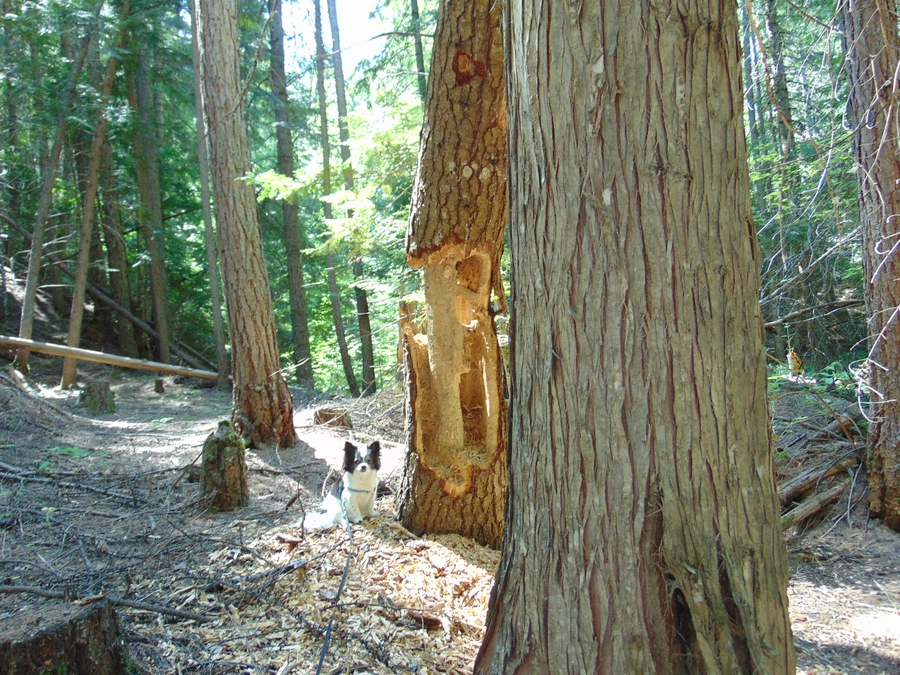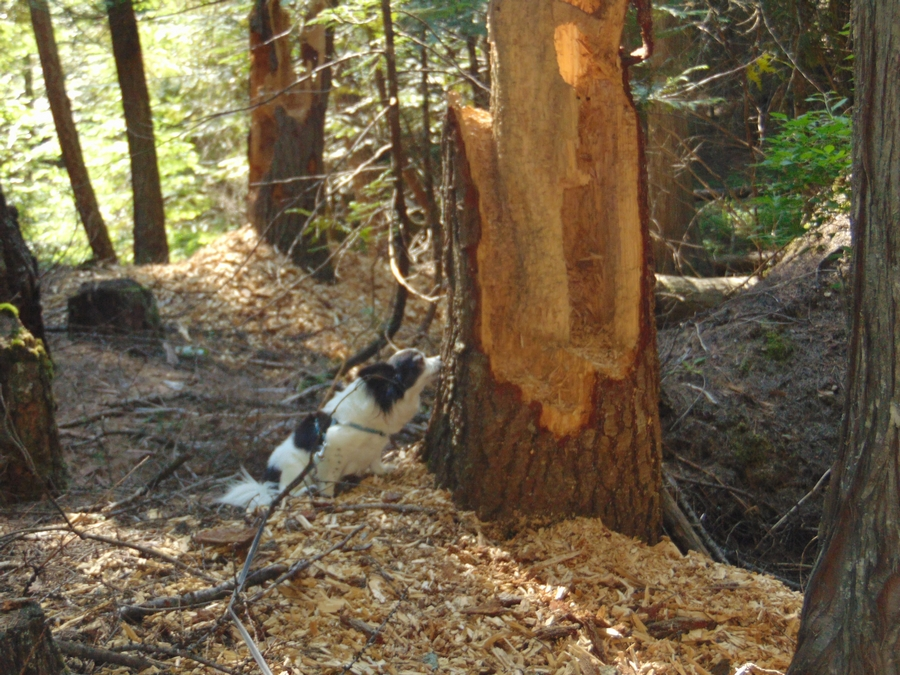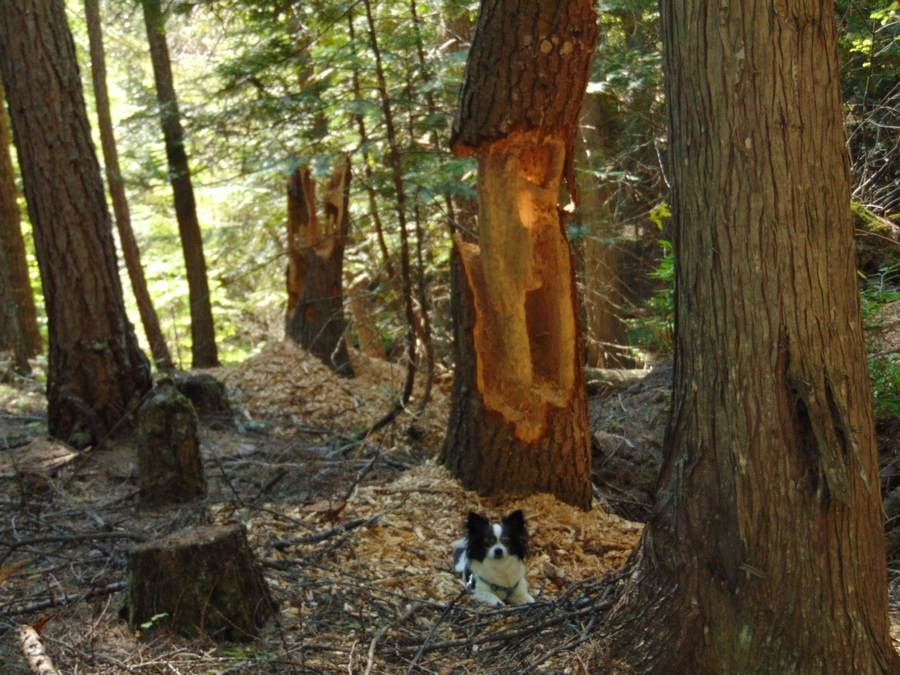it was hollow enough that i could of had Pixie pose inside a part of it but she gave me such a 'im scared im going to fall' look that i didnt make her pose in it
but she was happy to pose on the ground just fine :D

^^^^^^^^^^^^^^^^^^^^^^^^^^^^^^^^^^^^^^^^^^^^^^^^^^^^^^^^^^^^^^^^^^^^^^^^^^^^^^^^^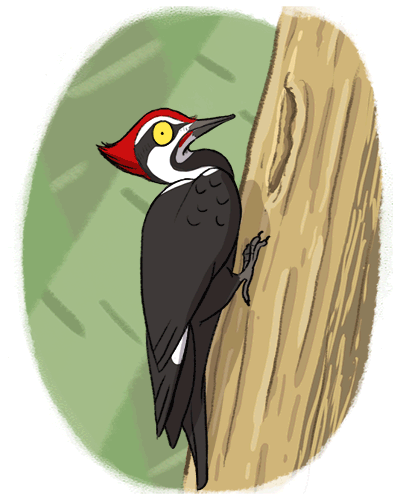Home   Back   Next

graphics from:
Gifcities